# SSLC Math's Solutions Chapter 3 Mathematics of Chance SCERT

Solutions for Mathematics SSLC Chapter 3: Mathematics of Chance. What does "Chance Mathematics" entail? First principles thinking, which is founded on logic and common sense obtained from precisely stated concepts, is the foundation of mathematics of chance (MoC). This answer explores the link between risk and probability in a daring way.

The course assessment and term evaluation themes are all covered in the PDF that is available on this website. Important questions and answers for chapter 3 of the mathematics of chance are also included in this PDF. In order to guarantee that students may achieve excellent scores on the SSLC examinations, both teachers and professionals have carefully devised the Mathematics of Chance.

In order to give you the greatest study materials that have been carefully screened and hand-selected by subject-matter experts faculty members from reputable schools around Kerala, the curriculum has been created utilising SCERT class 10 Math textbooks and previous year question papers.

 Board SCERT, Kerala Text Book SCERT Based Class SSLC Subject Maths Solution Chapter Chapter 3 Chapter Name Mathematics of Chance Category Kerala SSLC

## Kerala Syllabus SSLC Class 10 Maths Textbook Solution Chapter 3 Mathematics of Chance

### Chapter 3 Mathematics of Chance Textbook Solution

###### Questions Pg-69
Question 1.

A box contains 6 black and 4 white balls. If a ball is taken from it, what is the probability of it being black? And the probability of it being white?

Number of black balls in the box = 6

Number of white balls in the box = 4

Total numbers of balls in the box = 10

Probability of getting a black ball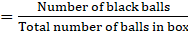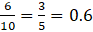Probability of getting a white ball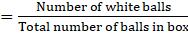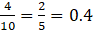Question 2.

There are 3 red balls and 7 green balls in a bag, 8 red and 7 green balls in another.

i) What is the probability of getting a red ball from the first bag?

ii) From the second bag?

iii) If all the balls are put in a single bag, what is the probability of getting a red ball from it?

Number of red balls in bag 1 = 3

Number of green balls in bag 1 = 7

Number of red balls in bag 2 = 8

Number of green balls in bag 2 = 7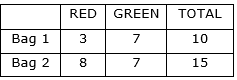i. Probability of getting red ball from first bag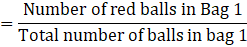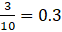ii. Probability of getting red ball from the second bag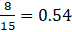iii. If balls are put together in one bag

Number of red balls = 3 + 8 = 11

Number of green balls = 7 + 7 = 14

Total number of balls = 25

Probability of getting red ball from the bag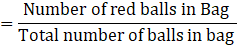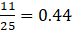Question 3.

One is asked to say a two-digit number. What is the probability of it being a perfect square?

Number of two-digit numbers = 90

Number of two-digit numbers which are perfect square = 6

Probability of being it perfect square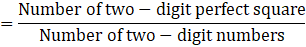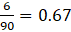∴ The probability of getting a two-digit perfect squared number is 0.67

Question 4.

Number from 1 to 50 are written on slips of paper and put in a box. A slip is to be drawn from it; but before doing so, one must make a guess about the number: either prime number or a multiple of five. Which is the better guess? Why?

Prime numbers between 1 – 50:

2, 3, 5, 7, 11, 13, 17, 19, 23, 29, 31, 37, 41, 43 and 47

Multiples of five:

5, 10, 15, 20, 25, 30, 35, 40, 45, 50

Number of prime number from 1 to 50 = 15

Number of multiples of 5 from 1 to 50 = 10

Total number of paper slips = 50

Probability of getting a prime number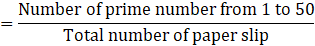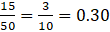Probability of getting a multiple of 5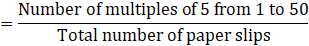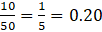From the calculated probabilities, we can observe that the probability of getting a paper slip which has prime number is 0.30 and the probability of getting a paper slip which has a multiple of 5 is 0.20.

Hence, choosing a prime number would be better because the probability of getting a prime number paper slip is higher than probability of getting a multiple of 5.

Question 5.

A bag contains 3 red beads and 7 green beads. Another contains one red and one green more. The probability of getting a red from which bag is more?

Number of red beads in bag 1 = 3

Number of green beads in bag 1 = 7

Number of red beads in bag 2 = 4

Number of green beads in bag 2 = 8

Total number of beads in bag 1 = 10

Total number of beads in bag 2 = 12

Probability of getting a red bead from bag 1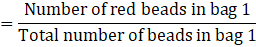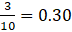Probability of getting a red bead from bag 2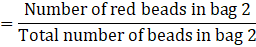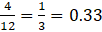∴ Probability of getting a red bead from bag 2 is higher.

###### Questions Pg-70
Question 1.

In each picture below, the explanation of the green part is given. Calculate in each, the probability of a dot put without looking to be within the green part.

A square got by joining the midpoints of a bigger square.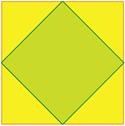The figure is given below: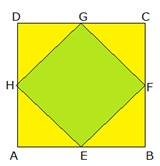Let side of bigger square be 2x

Then, AE = x

∴ Area of square = 4 × side

⇒ 4 × 2x

⇒ 8x

Area of Δ AHE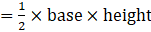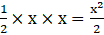∴ The area of yellow region = 4(Area of Δ AHE)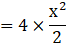= 2x2

Area of green region = Area of big square – Area of yellow region

⇒ 8x – 2x2

Probability of dot is putted in the green part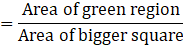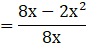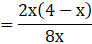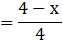Question 2.

In each picture below, the explanation of the green part is given. Calculate in each, the probability of a dot put without looking to be within the green part.

A square with all vertices on a circle.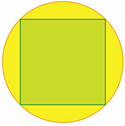The figure is shown below: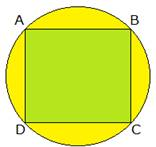Let the side of square be x

AC is the diameter of the circle as well as the diagonal of the square.

In Δ ABC

We know that, AB = BC (side of the square)

⇒ AB2 + BC2 = AC2 (Pythagoras theorem)

⇒ AB2 + AB2 = AC2

⇒ x2 + x2 = AC2

⇒ 2x2 = AC2

⇒ √2 x = ACArea of green region = Area of square = 4 × side

Area of green region = 4 × x

= 4x

Area of total region = Area of circle = πr2

Area of total region = π × (√2x)2 = 2πx2

Probability of getting dot inside green region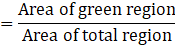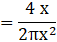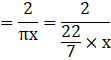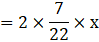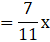=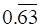Question 3.

In each picture below, the explanation of the green part is given. Calculate in each, the probability of a dot put without looking to be within the green part.

Circle exactly fitting inside a square.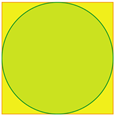The figure is shown below: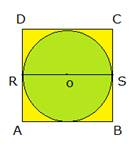Let the side of square be 2x

Also, RS = 2x

RS is also the diameter of the circle.

Radius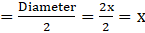Now,

Area of square = 4 × side

= 4 × 2x

= 8x

Area of green region(circle) = πr2

= π x2

Probability of dot is putted in the green part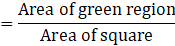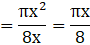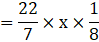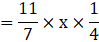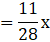= 0.40x (approx.)

Question 4.

In each picture below, the explanation of the green part is given. Calculate in each, the probability of a dot put without looking to be within the green part.

A triangle got by joining alternate vertices of a regular hexagon.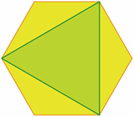The figure is shown below: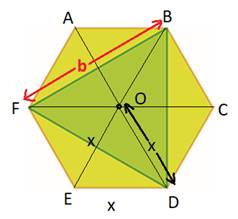Let the side of this hexagon be “x”.

Then, in this hexagon, a total of 6 equilateral triangles can be made, which are:

ΔOED, ΔODC, ΔOCB, ΔOBA, ΔOAF, ΔOFE.

Now, in the figure given below: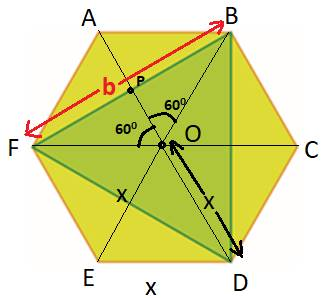In ΔOPF and ΔOPB,

∠POF = ∠POB (angle in equilateral triangle = 600)

OF = OB (sides of equilateral triangle)

OP is common in both the triangles.

Hence, we can say that ΔOPF ≅ ΔOPB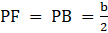Similarly, we can prove that ΔAPF ≅ Δ APB

⇒ AP = PO =Now in, right ΔOPF,

OP2 + PF2 = OF2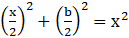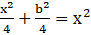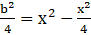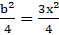⇒ b = √3x

Now,

Area of hexagon with side “x” =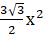Area of big triangle (green shaded) =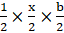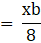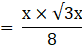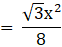Probability of dot is putted in the green part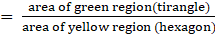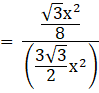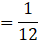= 0.083

Question 5.

In each picture below, the explanation of the green part is given. Calculate in each, the probability of a dot put without looking to be within the green part.

A regular hexagon formed by two overlapping equilateral triangles.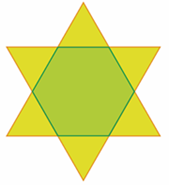The figure is given below: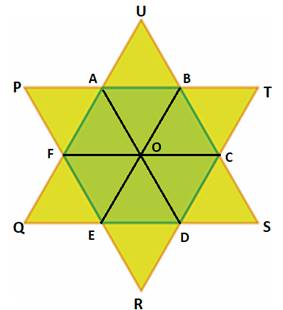Here,

The shaded area is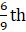of the big triangle.

Hence, the area will be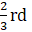of the bigger triangle.

Hence, the probability is 2/3.

###### Questions Pg-73
Question 1.

Rajani has three necklaces and three pairs of earrings, of green, blue and red stones. In what all different ways can she wear them? What is the probability of her wearing the necklace and earrings of the same colour? Of different colours?

Let the necklace and earrings of different stone be denoted by G, B and R for green, blue and red stones respectively.

Now,

Make pairs of necklace and earring like (necklace, earing)

Pairs are:

(G, G), (B, G), (R, G)

(G, B), (B, B), (R, B)

(G, R), (B, R), (R, R)

Total number of pairs = 9

Pairs having the same colour = 3

Probability of having same colour necklace and earing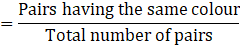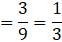Question 2.

A box contains four slips numbered 1, 2, 3, 4 and another box contains two slips numbered 1, 2. If one slip is taken from each, what is the probability of the sum of numbers being odd? What is the probability of the sum being even?

Let box 1 contains slip numbered 1, 2, 3 and 4 and box 2 contains slips numbered 1 and 2.

Let make pair of outcome from different boxes

i.e. (outcome of box 1, outcome of box 2)

Outcomes:

(1, 1), (2, 1), (3, 1), (4, 1)

(1, 2), (2, 2), (3, 2), (4, 2)

Total number of outcomes = 8

Outcomes having sum of the numbers as odd = 4

Outcomes having sum of the numbers as even = 4

Probability of the sum of numbers being odd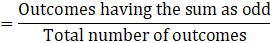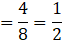= 0.5

Probability of the sum of numbers being even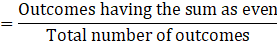= 0.5

Question 3.

A box contains four slips numbered 1, 2, 3, 4 and another contains three slips numbered 1, 2, 3. If one slip is taken from each, what is the probability of the product being odd? The probability of the product being even?

Let box 1 contains slip numbered 1, 2, 3 and 4 and box 2 contains slips numbered 1, 2 and 3.

Let make pair of outcome from different boxes

i.e. (outcome of box 1, outcome of box 2)

Outcomes:

(1, 1), (2, 1), (3, 1), (4, 1)

(1, 2), (2, 2), (3, 2), (4, 2)

(1, 3), (2, 3), (3, 3), (4, 3)

Total number of outcomes = 12

Outcomes having product of the numbers as odd = 4

Outcomes having product of the numbers as even = 8

Probability of the product of numbers being odd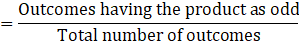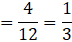= 0.33

Probability of the product of numbers being even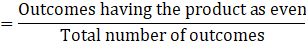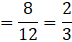= 0.67

Question 4.

From all two-digit numbers with either digit 1, 2, or 3 one number is chosen.

i) What is the probability of both digits being the same?

ii) What is the probability of the sum of the digits being 4?

Sample space:

11, 12, 13

21, 22, 23

31, 32, 33

i Total number of numbers formed = 9

Total number of numbers having same digit = 3

Probability of both digits being the same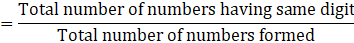= 0.33

ii Total number of numbers formed = 9

Total number of numbers sum being 4 = 3

Probability of both digits being the same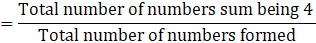= 0.33

Question 5.

A game for two players. First, each has to decide whether he wants odd number or even number. Then both raises some fingers of one hand. If the sum is odd, the one who chose odd at the beginning wins; if it is even, the one who chose even wins. In this game, which is the better choice at the beginning, odd or even?

Let us suppose who so ever wins gets point equal to the total sum of both players fingers.

There are two ways of playing, one who choose odd raises an odd number of fingers and one who choose even raises an even number of fingers or vice – a – versa.

There can be chances when both raises an odd or even.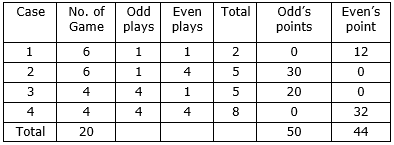So, in long run, odd is up to 6 points in 20 games.

∴ Choosing the odd would be a better option.

###### Questions Pg-76
Question 1.

In class 10A, there are 20 boys and 20 girls. In 10B, there are 15 boys and 25 girls. One student is to be selected from each class.

i) What is the probability of both being girls?

ii) What is the probability of both being boys?

iii) What is the probability of one boy and one girl?

iv) What is the probability of at least one boy?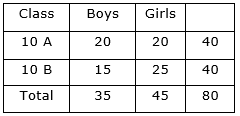Total number of students = 80

i. Probability of both being girl.

For both being a girl i.e. girl is selected from class 10A and another girl is selected from class 10B

Total number of girls = 45

Probability of both being girls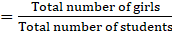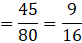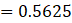ii. Probability of both being boys

For both being a boy i.e. boy is selected from class 10A and another boy is selected from class 10B

Total number of boys = 35

Probability of both being boys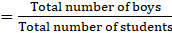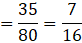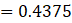iii. Probability of one boy and one girl

For one boy and one girl, a boy is selected from class 10A and a girl from class 10B or vice a versa.

i.e. P (boy from class 10A) × P (girl from class 10B) +

P (girl from class 10A) × P (boy from class 10B)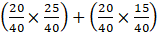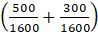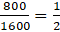= 0.50

Probability of one boy and one girl is 0.50.

iv. Probability of at least one boy.

For at least one boy means one boy and another girl, or both boy.

In this, one boy and other girl can occur in two ways:

1st boy and 2nd girl or the other way round.

(20 × 25) + (20 × 15)

= 500 + 300

= 800

∴ the possibility is 0.50 (obtained in previous part)

We have already seen the possibility of both the boys in above solved parts i.e. probability of both boys is 0.4375

∴ Probability of at least one boy = 0.50 + 0.4375

= 0.9375

Question 2.

One is asked to say a two-digit number.

i) What is the probability of both digits being the same?

ii) What is the probability of the first digit being larger?

iii) What is the probability of the first digit being smaller?

Total number of two -digit numbers = 90

i. Probability of both digits being the same

Total number of numbers having same digit = 9

Probability of both digits being same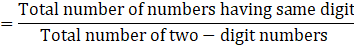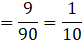= 0.10

ii. Probability of first digit being larger

Total number of numbers having first digit larger = 45

10, 20, 21, 30, 31, 32, 40, 41, 42, 43, 50, 51, 52, 53, 54, 60, 61, 62, 63, 64, 65, 70, 71, 72, 73, 74, 75, 76, 80, 81, 82, 83, 84, 85, 86, 87, 90, 91, 92, 93, 94, 95, 96, 97, 98.

Probability of first digit being larger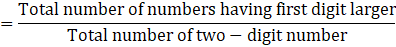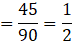= 0.50

iii. Probability of first digit being smaller

Total number of numbers having first digit smaller = 36

12, 13, 14, 15, 16, 17, 18, 19, 23, 24, 25, 26, 27, 28, 29, 34, 35, 36, 37, 38, 39, 45, 46, 47, 48, 49, 56, 57, 58, 59, 67, 68, 69, 78, 79, 89.

Probability of first digit being smaller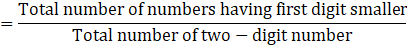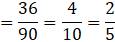= 0.25

Question 3.

Two dice with faces numbered from 1 to 6 are rolled together. What are the possible sums? Which of these sums has the maximum probability?

Sample space of two dice rolled together:

(1, 1) (1, 2) (1, 3) (1, 4) (1, 5) (1, 6)

(2, 1) (2, 2) (2, 3) (2, 4) (2, 5) (2, 6)

(3, 1) (3, 2) (3, 3) (3, 4) (3, 5) (3, 6)

(4, 1) (4, 2) (4, 3) (4, 4) (4, 5) (4, 6)

(5, 1) (5, 2) (5, 3) (5, 4) (5, 5) (5, 6)

(6, 1) (6, 2) (6, 3) (6, 4) (6, 5) (6, 6)

Maximum sum will be 12 and minimum will be 2

Sum (2) – {(1, 1)} = 1

Sum (3) – {(1, 2) (2, 1)} = 2

Sum (4) – {(1, 3) (2, 2) (3, 1)} = 3

Sum (5) – {(1, 4) (2, 3) (3, 2) (4, 1)} = 4

Sum (6) – {(1, 5) (2, 4) (3, 3) (4, 2) (5, 1)} = 5

Sum (7) – {(1, 6) (2, 5) (3, 4) (4, 3) (5, 2) (6, 1)} = 6

Sum (8) – {(2, 6) (3, 5) (4, 4) (5, 3) (6, 2)} = 5

Sum (9) – {(3, 6) (4, 5) (5, 4) (6, 3)} = 4

Sum (10) – {(4, 6) (5, 5) (6, 4)} = 3

Sum (11) – {(5, 6) (6, 5)} = 2

Sum (12) – {(6, 6)} = 1

Sum of 6 has maximum possibility because it has more number of outcomes.

### SSLC Maths Textbook Solution

Feel free to comment and share this article if you found it useful. Give your valuable suggestions in the comment session or contact us for any details regarding the HSE Kerala SSLC class 10 syllabus, Previous year question papers, and other study materials.